# Variational inequalities

The variational formulations (also called weak formulations) of many non-linear boundary value problems result in variational inequalities rather than variational equations. Analogously to partial differential equations, variational inequalities can be of elliptic, parabolic, hyperbolic, etc. type.

Suppose thatis a Banach space,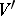is its dual and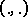is the duality pairing betweenand. Let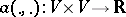be a continuous, bilinear, coercive or semi-coercive form (cf. also Coerciveness inequality), let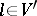, and letbe a non-empty, convex, closed subset. Moreover, let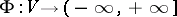,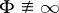, be a convex, lower semi-continuous functional on.

Then an elliptic variational inequality usually has one of the following two forms:

1) Find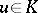such that2) Find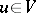such that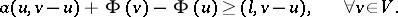Eigenvalue problems related to variational inequalities have been formulated and studied, as well as optimal control problems of system governed by, i.e. having as state relations, variational inequalities. In mechanics, variational inequalities arise in a natural way because they are expressions of the principle of virtual work or power in inequality form. The mathematical study of variational inequalities began in the early 1960s with the work of G. Fichera, J.L. Lions and G. Stampacchia [a1], [a2]. J.J. Moreau [a3] and H. Brézis [a4] connected the theory of variational inequalities to convex analysis, especially to the notion of subdifferentiability, and to the theory of maximal monotone operators [a5]. For non-coercive variational inequalities see [a6], [a7]; for their numerical study, see [a8], [a9]. Eigenvalue problems for variational inequalities can be found in [a10], while applications can be found in [a11], [a12], where also their relation to convex non-smooth energy minimization problems is presented. For the optimal control problem of systems governed by variational inequalities see [a13].

How to Cite This Entry:
Variational inequalities. P.D. Panagiotopoulos (originator), Encyclopedia of Mathematics. URL: http://encyclopediaofmath.org/index.php?title=Variational_inequalities&oldid=12608
This text originally appeared in Encyclopedia of Mathematics - ISBN 1402006098